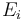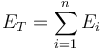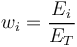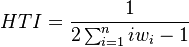# Hall-Tideman Index

## Definition

The Hall-Tideman index is a metric useful for the purpose of measuring Concentration Risk diversity or inequality metrics. An alternative name is Rosenbluth Index.

## Details

More precisely, if we have n values$E_i$ summing up to a total of$E_T = \sum^{n}_{i=1} E_{i}$

and each value fraction is defined as$w_{i} = \frac{E_i}{E_T}$

Then the HTI index is defined as$HTI = \frac{1}{2 \sum^{n}_{i=1} i w_{i} - 1}$